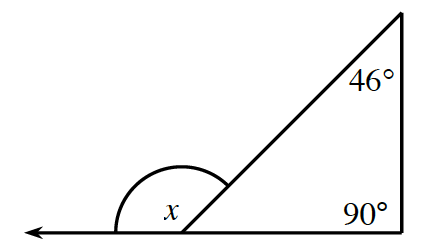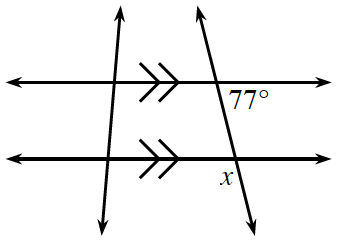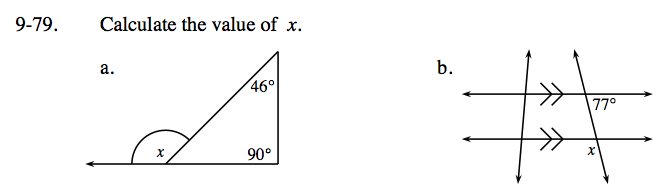Home > CC3 > Chapter 9 > Lesson 9.2.2 > Problem9-79

9-79.
1. Calculate the value of x. Homework Help ✎

1.2.An exterior angle of a triangle is equal to the sum of the two remote interior angles.

x = 46 + 90

Notice that the 77° angle corresponds to the angle adjacent to angle x. This angle is complementary to angle x.

The vertical angle is equal to the 77° angle because they are alternate interior angles on parallel lines.